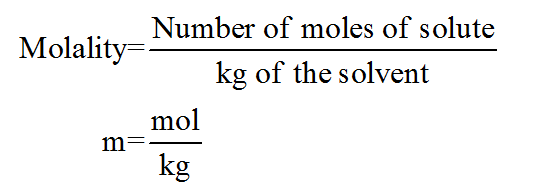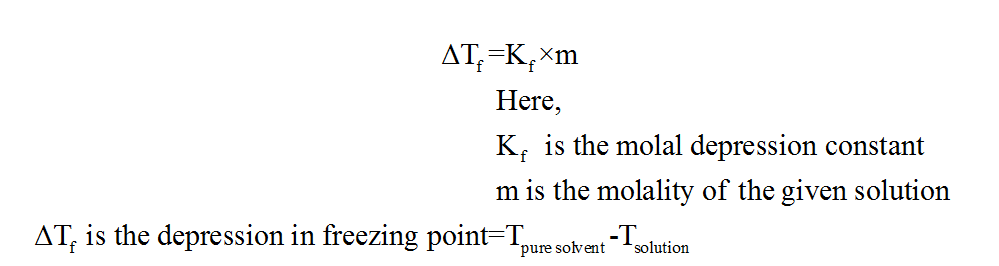# Calculate the freezing point of a solution of 35 g methyl salicylate dissolved in 800 g of benzene for benzene is 5.10 Celsius/m and the freezing point is 5.50 degrees Celsius for benzene

Question
1 views

Calculate the freezing point of a solution of 35 g methyl salicylate dissolved in 800 g of benzene for benzene is 5.10 Celsius/m and the freezing point is 5.50 degrees Celsius for benzene

check_circle

Step 1

The term molality refers to the ratio of number of moles of the given solute to the amount of solvent in kilogram. The formula is as follows:Step 2

The freezing point can be defined as the temperature at which a given liquid changes into the solid form.

Step 3

The relation between the freezing point depression and molality is shown below:...

### Want to see the full answer?

See Solution

#### Want to see this answer and more?

Solutions are written by subject experts who are available 24/7. Questions are typically answered within 1 hour.*

See Solution
*Response times may vary by subject and question.
Tagged in

### Chemistry